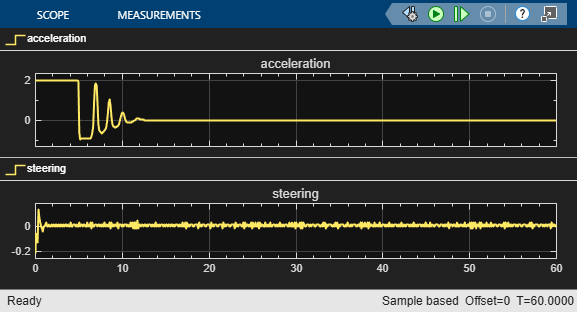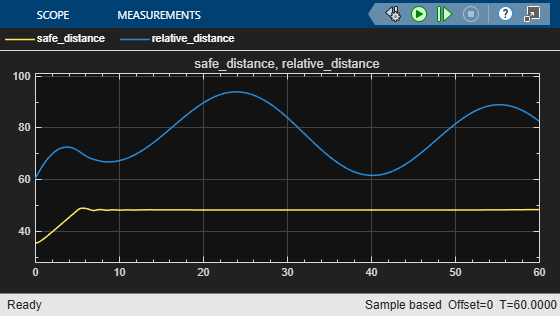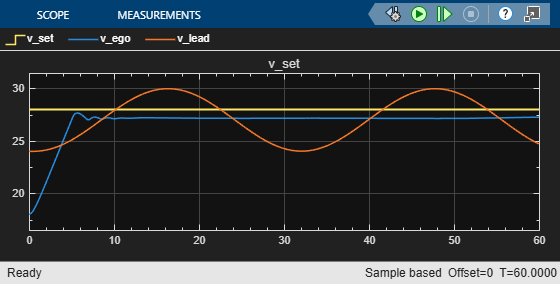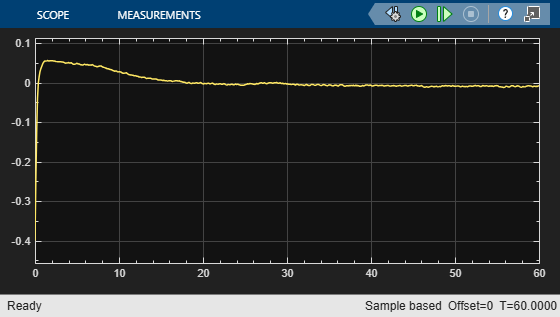# Train Multiple Agents for Path Following Control

This example shows how to train multiple agents to collaboratively perform path-following control (PFC) for a vehicle. The goal of PFC is to make the ego vehicle travel at a set velocity while maintaining a safe distance from a lead car by controlling longitudinal acceleration and braking, and also while keeping the vehicle travelling along the centerline of its lane by controlling the front steering angle. For more information on PFC, see Path Following Control System (Model Predictive Control Toolbox).

### Overview

An example that trains a reinforcement learning agent to perform PFC is shown in Train DDPG Agent for Path-Following Control. In that example, a single deep deterministic policy gradient (DDPG) agent is trained to control both the longitudinal speed and lateral steering of the ego vehicle. In this example, you train two reinforcement learning agents — A DDPG agent provides continuous acceleration values for the longitudinal control loop and a deep Q-network (DQN) agent provides discrete steering angle values for the lateral control loop.

The trained agents perform PFC through cooperative behavior and achieve satisfactory results.

### Create Environment

The environment for this example includes a simple bicycle model for the ego car and a simple longitudinal model for the lead car. The training goal is to make the ego car travel at a set velocity while maintaining a safe distance from lead car by controlling longitudinal acceleration and braking, while also keeping the ego car travelling along the centerline of its lane by controlling the front steering angle.

`multiAgentPFCParams`

```mdl = "rlMultiAgentPFC"; open_system(mdl)```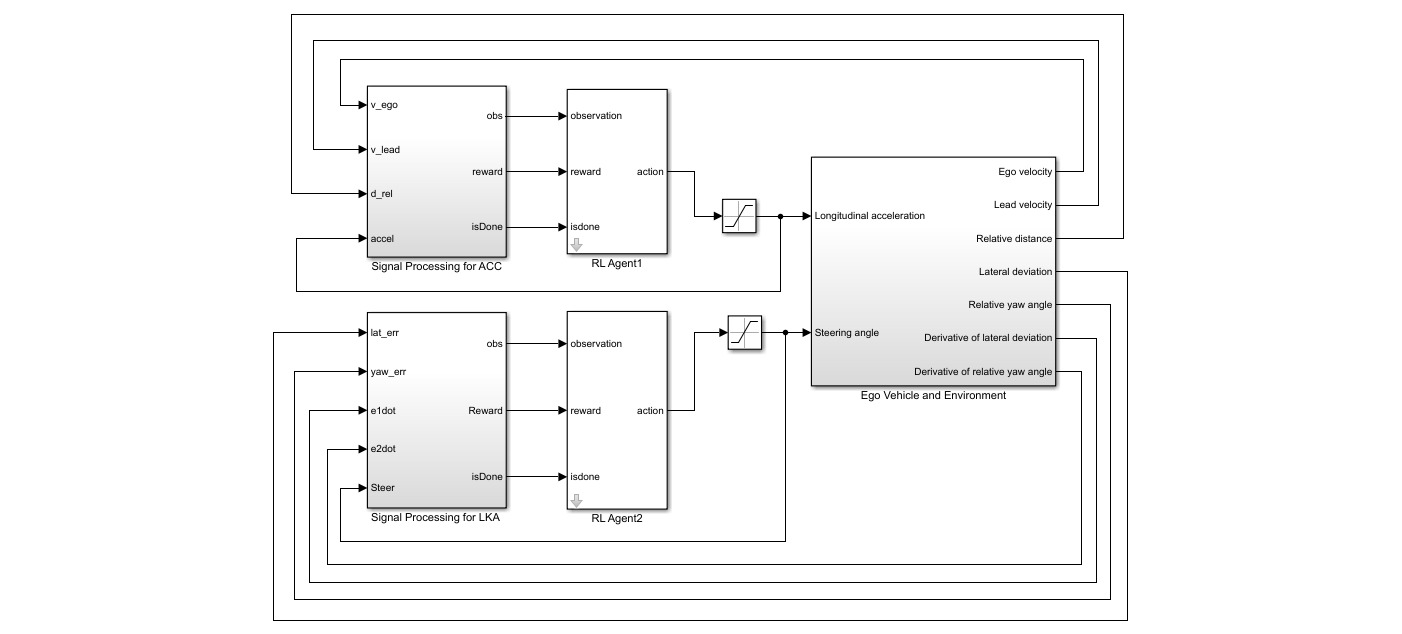In this model, the two reinforcement learning agents (RL Agent1 and RL Agent2) provide longitudinal acceleration and steering angle signals, respectively.

The simulation terminates when any of the following conditions occur.

• $|{e}_{1}|>1$ (magnitude of the lateral deviation exceeds 1)

• ${V}_{ego}<0.5$ (longitudinal velocity of the ego car drops below 0.5.

• ${D}_{rel}<0$ (distance between the ego and lead car is below zero)

For the longitudinal controller (RL Agent1):

• The reference velocity for the ego car ${V}_{ref}$ is defined as follows. If the relative distance is less than the safe distance, the ego car tracks the minimum of the lead car velocity and driver-set velocity. In this manner, the ego car maintains some distance from the lead car. If the relative distance is greater than the safe distance, the ego car tracks the driver-set velocity. In this example, the safe distance is defined as a linear function of the ego car longitudinal velocity $\mathit{V}$, that is, ${t}_{gap}*V+{D}_{default}$. The safe distance determines the tracking velocity for the ego car.

• The observations from the environment contain the longitudinal measurements: the velocity error ${e}_{V}={V}_{ref}-V$, its integral $\int e$, and the ego car longitudinal velocity $\mathit{V}$.

• The action signal consists of continuous acceleration values between -3 and 2 m/s^2.

• The reward ${\mathit{r}}_{\mathit{t}}$, provided at every time step $\mathit{t}$, is

`${r}_{t}=-\left(10{e}_{V}^{2}+100{a}_{t-1}^{2}\right)×0.001-10{F}_{t}+{M}_{t}$`

Here, ${a}_{t-1}$ is the acceleration input from the previous time step, and:

• ${F}_{t}=1$ if the simulation is terminated, otherwise ${F}_{t}=0$.

• ${M}_{t}=1$ if ${e}_{V}^{2}<1$, otherwise ${M}_{t}=0$.

For the lateral controller (RL Agent2):

• The observations from the environment contain the lateral measurements: the lateral deviation ${\mathit{e}}_{1}$, relative yaw angle ${\mathit{e}}_{2}$, their derivatives ${\underset{}{\overset{˙}{e}}}_{1}$ and ${\underset{}{\overset{˙}{e}}}_{2}$, and their integrals $\int {\mathit{e}}_{1}$ and $\int {\mathit{e}}_{2}$.

• The action signal consists of discrete steering angle actions which take values from -15 degrees (-0.2618 rad) to 15 degrees (0.2618 rad) in steps of 1 degree (0.0175 rad).

• The reward ${\mathit{r}}_{\mathit{t}}$, provided at every time step $\mathit{t}$, is

`${r}_{t}=-\left(100{e}_{1}^{2}+500{u}_{t-1}^{2}\right)×0.001-10{F}_{t}+2{H}_{t}$`

Here, ${u}_{t-1}$ is the steering input from the previous time step, ${a}_{t-1}$ is the acceleration input from the previous time step, and:

• ${F}_{t}=1$if the simulation is terminated, otherwise ${F}_{t}=0$.

• ${H}_{t}=1\text{}$ ${e}_{1}^{2}<0.01\text{}$, otherwise ${H}_{t}=0\text{}$.

The logical terms in the reward functions (${F}_{t}$, ${M}_{t}$, and ${H}_{t}$) penalize the agents if the simulation terminates early, while encouraging the agents to make both the lateral error and velocity error small.

Create the observation and action specifications for longitudinal control loop.

```obsInfo1 = rlNumericSpec([3 1]); actInfo1 = rlNumericSpec([1 1],LowerLimit=-3,UpperLimit=2);```

Create the observation and action specifications for lateral control loop.

```obsInfo2 = rlNumericSpec([6 1]); actInfo2 = rlFiniteSetSpec((-15:15)*pi/180);```

Combine the observation and action specifications as a cell array.

```obsInfo = {obsInfo1,obsInfo2}; actInfo = {actInfo1,actInfo2};```

Create a Simulink environment interface, specifying the block paths for both agent blocks. The order of the block paths must match the order of the observation and action specification cell arrays.

```blks = mdl + ["/RL Agent1", "/RL Agent2"]; env = rlSimulinkEnv(mdl,blks,obsInfo,actInfo);```

Specify a reset function for the environment using the `ResetFcn` property. The function `pfcResetFcn` randomly sets the initial poses of the lead and ego vehicles at the beginning of every episode during training.

`env.ResetFcn = @pfcResetFcn;`

### Create Agents

For this example you create two reinforcement learning agents. First, fix the random seed for reproducibility.

`rng(0)`

Both agents operate at the same sample time in this example. Set the sample time value (in seconds).

`Ts = 0.1;`

#### Longitudinal Control

The agent for the longitudinal control loop is a DDPG agent. A DDPG agent approximates the long-term reward given observations and actions using a critic value function representation and selects actions using an actor policy representation. For more information on creating deep neural network value function and policy representations, see Create Policies and Value Functions.

Use the `createCCAgent` function to create a DDPG agent for longitudinal control. The structure of this agent is similar to the Train DDPG Agent for Adaptive Cruise Control example.

`agent1 = createACCAgent(obsInfo1,actInfo1,Ts);`

#### Lateral Control

The agent for the lateral control loop is a DQN agent. A DQN agent approximates the long-term reward given observations and actions using a critic value function representation.

Use the `createLKAAgent` function to create a DQN agent for lateral control. The structure of this agent is similar to the Train DQN Agent for Lane Keeping Assist example.

`agent2 = createLKAAgent(obsInfo2,actInfo2,Ts);`

### Train Agents

Specify the training options. For this example, use the following options.

• Run each training episode for at most 5000 episodes, with each episode lasting at most `maxsteps` time steps.

• Display the training progress in the Episode Manager dialog box (set the `Verbose` and `Plots` options).

• Stop training the DDPG and DQN agents when they receive an average reward greater than 480 and 1195, respectively. When one agent reaches its stop criteria, it simulates its own policy without learning while the other agent continues training.

```Tf = 60; % simulation time maxepisodes = 5000; maxsteps = ceil(Tf/Ts); trainingOpts = rlTrainingOptions(... MaxEpisodes=maxepisodes,... MaxStepsPerEpisode=maxsteps,... Verbose=false,... Plots="training-progress",... StopTrainingCriteria="AverageReward",... StopTrainingValue=[480,1195]);```

Train the agents using the `train` function. Training these agents is a computationally intensive process that takes several minutes to complete. To save time while running this example, load a pretrained agent by setting `doTraining` to `false`. To train the agent yourself, set `doTraining` to `true`.

```doTraining = false; if doTraining % Train the agent. trainingStats = train([agent1,agent2],env,trainingOpts); else % Load pretrained agents for the example. load("rlPFCAgents.mat") end```

The following figure shows a snapshot of the training progress for the two agents.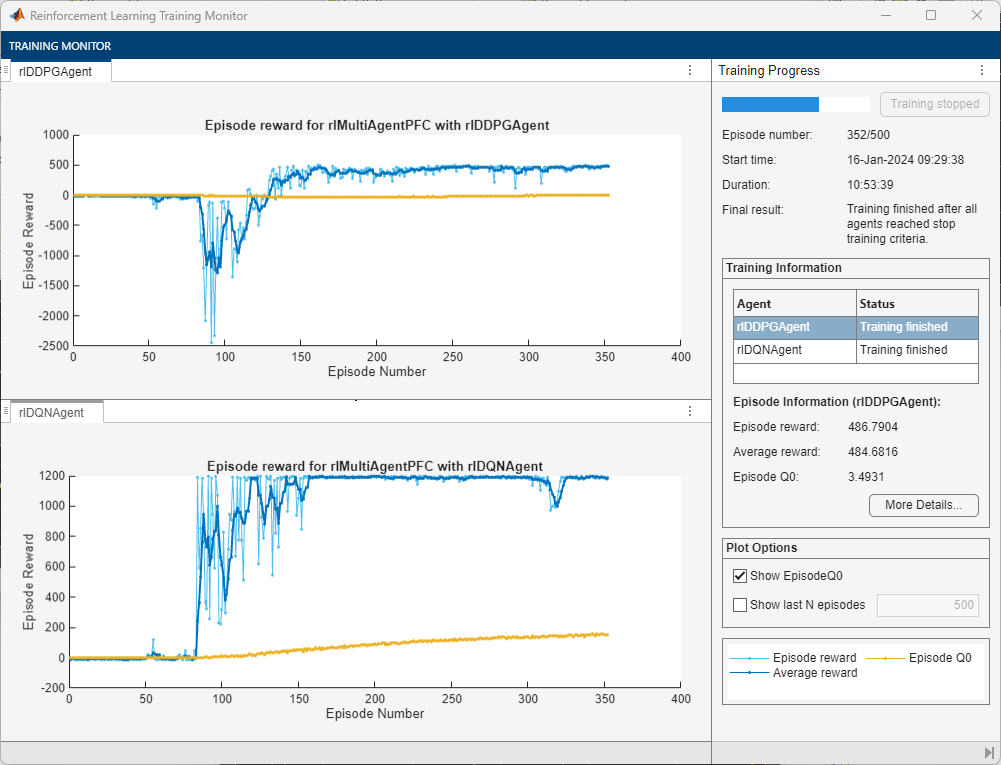### Simulate Agents

To validate the performance of the trained agents, simulate the agents within the Simulink environment by uncommenting the following commands. For more information on agent simulation, see `rlSimulationOptions` and `sim`.

```% simOptions = rlSimulationOptions(MaxSteps=maxsteps); % experience = sim(env,[agent1, agent2],simOptions);```

To demonstrate the trained agent using deterministic initial conditions, simulate the model in Simulink.

```e1_initial = -0.4; e2_initial = 0.1; x0_lead = 80; sim(mdl)```

The following plots show the results when the lead car is 70 m ahead of the ego car at the beginning of simulation.

• The lead car changes speed from 24 m/s to 30 m/s periodically (top-right plot). The ego car maintains a safe distance throughout the simulation (bottom-right plot).

• From 0 to 30 seconds, the ego car tracks the set velocity (top-right plot) and experiences some acceleration (top-left plot). After that, the acceleration is reduced to 0.

• The bottom-left plot shows the lateral deviation. As shown in the plot, the lateral deviation is greatly decreased within 1 second. The lateral deviation remains less than 0.1 m.